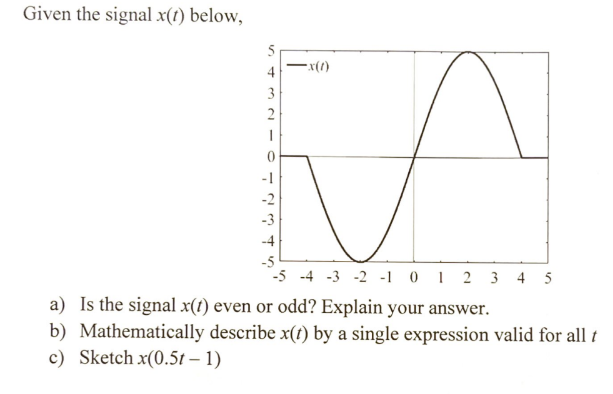# (Solved): Given the signal \( x(t) \) below, a) Is the signal \( x(t) \) even or odd? Explain your answer. b ...Given the signal \( x(t) \) below, a) Is the signal \( x(t) \) even or odd? Explain your answer. b) Mathematically describe \( x(t) \) by a single expression valid for all \( t \) c) Sketch \( x(0.5 t-1) \)

We have an Answer from Expert